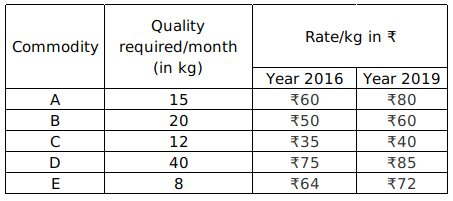Question 73

# The following table gives the details of Five commodities A, B, C, D and E with quantity required and their costs for a family in a month. Study the table and answer the questions that follow.The ratio of the total amount spent on A and D commodities in the year 2019 is:

Solution

Total amount spent on A commodities in the year 2019 = quantity $$\times rate = 15 \times 80$$

Total amount spent on D commodities in the year 2019 = $$40 \times 85$$

The ratio of the total amount spent on A and D commodities in the year 2019 = $$15 \times 80 : 40 \times 85 = 6 : 17$$